### Home > CALC > Chapter 10 > Lesson 10.1.3 > Problem10-20

10-20.
1. Evaluate each limit below. Homework Help ✎

1.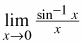2.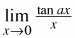3.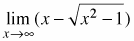4.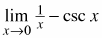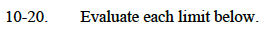Use l'Hôpital's Rule.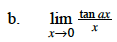Use l'Hôpital's Rule.
Think of a as a number.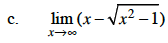Make the expression a fraction over 1, then rationalize the numerator.
The resulting expression can be used to evaluate the limit.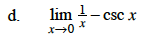$=\lim_{x\to 0}\Big(\frac{1}{x}-\frac{1}{\sin(x)}\Big)$

$=\lim_{x\to 0}\frac{\sin(x)-x}{x\sin(x)}$

Use two iterations of l'Hôpital's Rule.